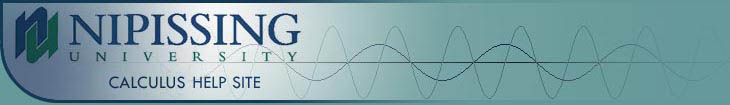TUTORIALS
TUTORIALS HOME

GENERAL MATH
NUMBER SETS
ABSOLUTE VALUE & INEQUALITIES
SETS & INTERVALS
FRACTIONS
POLYNOMIALS
LINEAR EQUATIONS
GEOMETRY
FINITE SERIES
TRIGONOMETRY
EXPONENTS
LOGARITHMS
INDUCTION

CALCULUS
LIMITS
DERIVATIVES
RELATED RATES & OPTIMIZATION
CURVE SKETCHING
INTEGRALS
AREA & VOLUME
INVERSE FUNCTIONS

MAIN
HOME
TESTS
TUTORIALS
SAMPLE PROBLEMS
COMMON MISTAKES
STUDY TIPS
GLOSSARY
CALCULUS APPLICATIONS
MATH HUMOUR

# Tangents and Limits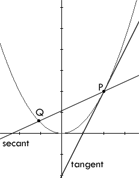A tangent to a curve is a straight line that touches the curve at a single point but does not intersect it at that point. For example, in the figure to the right, the y-axis would not be considered a tangent line because it intersects the curve at the origin. A secant to a curve is a straight line that intersects the curve at two or more points.

In the figure to the right, the tangent line intersects the curve at a single point P but does not intersect the curve at P. The secant line intersects the curve at points P and Q.

The concept of limits begins with the tangent line problem. We want to find the equation of the tangent line to the curve at the point P. To find this equation, we will need the slope of the tangent line. But how can we find the slope when we only know one point on the line? The answer is to look at the slope of the secant line. It's slope can be determined quite easily since there are two known points P and Q. As you slide the point Q along the curve, towards the point P, the slope of the secant line will become closer to the slope of the tangent line. Eventually, the point Q will be so close to P, that the slopes of the tangent and secant lines will be approximately equal.

A limit of a function is written as :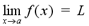We want to find the limit of f(x) as x approaches a. To do this, we try to make the values of f(x) close to the limit L, by taking x values that are close to, but not equal to, a. In short, f(x) approaches L as x approaches a.

# Examples

1 | Find the equation of the tangent line to the curve at point P

# Left and Right Hand Limits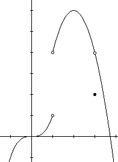The previous example shows that the value a can be approached from both the left and right sides. Each side has its own limit. For example, as x approaches a from the right side we have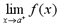and as x approaches a from the left we have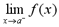.

The graph to the right shows an example of a function with different right and left hand limits at the point x = 1. As x approaches 1 from the left side, the limit of f(x) approaches 1. As x approaches 1 from the right side however, the limit of f(x) approaches 4. In this case, the limit of f(x) as x approaches 1 does not exist, because the left and right hand limits do not approach the same value. This idea leads to the following theorem: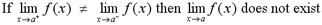For the limit to exist, the left and right hand limits must approach the same value. In our example, as x approaches 3, the left and right hand limits both approach a value of 4. Since the left and right hand limits are the same, the limit of f(x) as x approaches 3 exists and is equal to 4. Even though the actual value of f(3) is equal to 2, the limit is equal to 4. This gives the following theorem: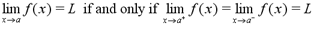# Examples

2 | Evaluate the limit, if it exists

Note: The example above uses basic concepts that are covered in the sections below.

# Infinite Limits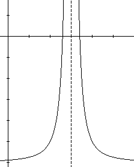If a function is defined on either side of a, but the limit as x approaches a is infinity or negative infinity, then the function has an infinite limit. The graph of the function will have a vertical asymptote at a. A curve y=f(x) will have a vertical asymptote at x = a if any of the following conditions hold: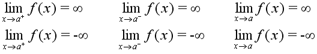Note: When dealing with rational functions, often there is a vertical asymptote at values of x that make the denominator equal to 0. However, you must still show that one of the conditions above holds true to prove that there is a vertical asymptote at that point. For example, function f(x)=(x2-1)/(x-1) is undefined at x=1 but does not have a vertical asymptote at x=1. In fact, f(x) = x+1 for all x1.

# Examples

3 | Find the vertical asymptotes of the function

# Laws of Limits

Calculating limits using graphs and tables takes a lot of unnecessary time and work. Using the limit laws listed below, limits can be calculated much more quickly and easily.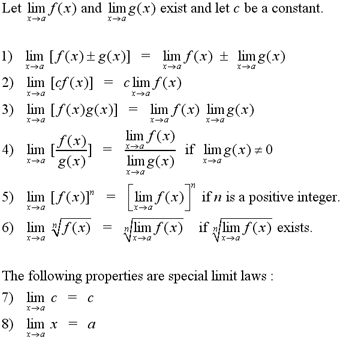From the limit laws above, comes the property of direct substitution. This property makes it possible to solve most rational and polynomial functions. The property of direct substitution states: For any rational or polynomial function f, if a is in the domain of f then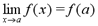Often, the method of direct substitution cannot be used because a is not in the domain of f. In these cases, it is sometimes possible to factor the function and eliminate terms so that the function is defined at the point a. For an example of factoring, see example 6 below.

# Examples

4 | Evaluate the limit using limit laws
5 | Evaluate the limit using the property of direct substitution
6 | Evaluate the limit by factoring f(x) and eliminating terms

# The Squeeze Theorem

The squeeze theorem is an important concept that will be very helpful in upper year calculus courses. The squeeze theorem states: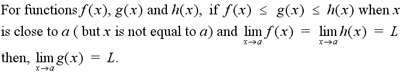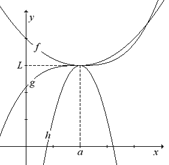In simpler terms, the squeeze theorem states that if the graph of g is squeezed between the graphs of f and h when x is near a, and if f and h have the same limit L as x approaches a, then the limit of g as x approaches a is also L. The graph to the right illustrates the squeeze theorem.

Note: The function g does not have to be completely contained between f and h. It must only be contained between f and h while x is near a. The graph illustrates where the functions f and g cross each other.

# Examples

7 | Evaluate the limit using the squeeze theorem

# Continuity and Discontinuity

A function f is continuous at a number a if: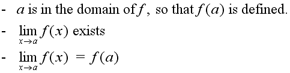Note: When proving that a function is continuous, you may only show that the limit of f(x) as x approaches a is equal to f(a). This property implies that f(a) is defined and that the limit exists.

A function is continuous on an interval if it is continuous at every number that falls within that interval. Continuous functions have the following properties for simple operations. If functions f and g are continuous at a, and c is a constant, then the following functions will also be continuous at a: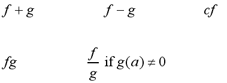A function is discontinuous at a if it is defined near a but not continuous at a. To prove that a function is discontinous, we must show which of the requirements of continuous functions that it fails to hold for. There are several different types of discontinuity, which are listed below.

Removable discontinuity: A function has a removable discontinuity at a if the limit as x approaches a exists, but either f(a) is different from the limit or f(a) does not exist. It is called removable discontuniuity because the discontinuity can be removed by redefining the function so that it is continuous at a. In example #6 above, the function has a removable discontinuity at x = 3 because if the function is redefined so that f(3) = -4/7, it will be continuous at x = 3.

Infinite discontinuity: A function has an infinite discontinuity at a if the limit as x approaches a is infinite. In example #3 above, the function has an infinite discontinuity at every point a = k*pi, since each point has an infinite limit.

Jump discontinuity: A function has a jump discontinuity at a if the left- and right-hand limits as x approaches a exist, but are different. It is called jump discontinuity because the function jumps from the left-hand limit to the right-hand limit at each point. In example #2 above, the function has a jump discontinuity at x = 0, since the right and left hand limits approach different values.

Note: Polynomial functions are continuous everywhere. Rational, root and trigonometric functions are continuous at every number in their domain. In other words, they are continuous wherever they are defined.

# Examples

8 | Determine where the function is continuous
9 | Explain why the function is discontinuous at each point

# The Intermediate Value Theorem

The intermediate value theorem states: If f is a continuous function on the closed interval [a, b] and N is a number between f(a) and f(b), where f(a) does not equal f(b), then there exists a number c in interval (a, b) such that f(c) = N.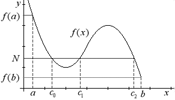The intermediate value theorem simply says that if a function is continuous on a closed interval [a, b], and f(a) is different from f(b), then every value on the y-axis between f(a) and f(b) has a corresponding value on the x-axis between a and b.

Note: As the graph to the right illustrates, there can be more than one value c in (a, b) for a number N betwen f(a) and f(b). The graph shows that there exist 3 numbers, c0, c1 and c2, such that f(c0)=f(c1)=f(c2)=N.

The intermediate value theorem is helpful when proving that a root of a function exists in a certain interval.

# Examples

10 | Show that the function has a root between a and b

# Tangents and Limits (Revisited)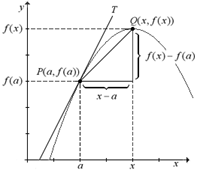As explained at the beginning of this tutorial, a tangent to a curve is a line that touches the curve at a single point, P(a,f(a)). The tangent line T is the line through the point P with the slope :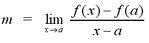given that this limit exists. The graph to the right illustrates how the slope of the tangent line is derived. The slope of the secant line PQ is given by f(x)-f(a)/x-a. As x approaches a, the slope of PQ becomes closer to the slope of the tangent line T. If we take the limit of the slope of the secant line as x approaches a, it will be equal to the slope of the tangent line T.

The slope of the tangent line becomes much easier to calculate if we consider the following conditions. If we let the distance between x and a be h, so that x=a+h, and substitute that equality for x in the slope formula, we get: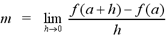Note: Either of the limit formulas above can be used to find the slope. You will obtain the same answer using either formula.

These formulas have many practical applications. They can be used to find the instantaneous rates of change of variables. For example, if we use the formula above, the instantaneous velocity at time t=a is equal to the limit of f(a+h)-f(a)/h as h approaches 0.

# Examples

11 | Find the equation of the tangent line to the curve at the point P
12 | Find the instantaneous rate of change

For more practice with the concepts covered in the limits tutorial, visit the Limit Problems page at the link below. The solutions to the problems will be posted after the limits chapter is covered in your calculus course.

To test your knowledge of limits, try taking the general limits test on the iLrn website or the advanced limits test at the link below.

Limit Problems
General Limit Test on iLrn

| Top of Page |

COURSE HOMEPAGES
MATH 1036
MATH 1037

FACULTY HOMEPAGES
Alex Karassev
Ted Chase